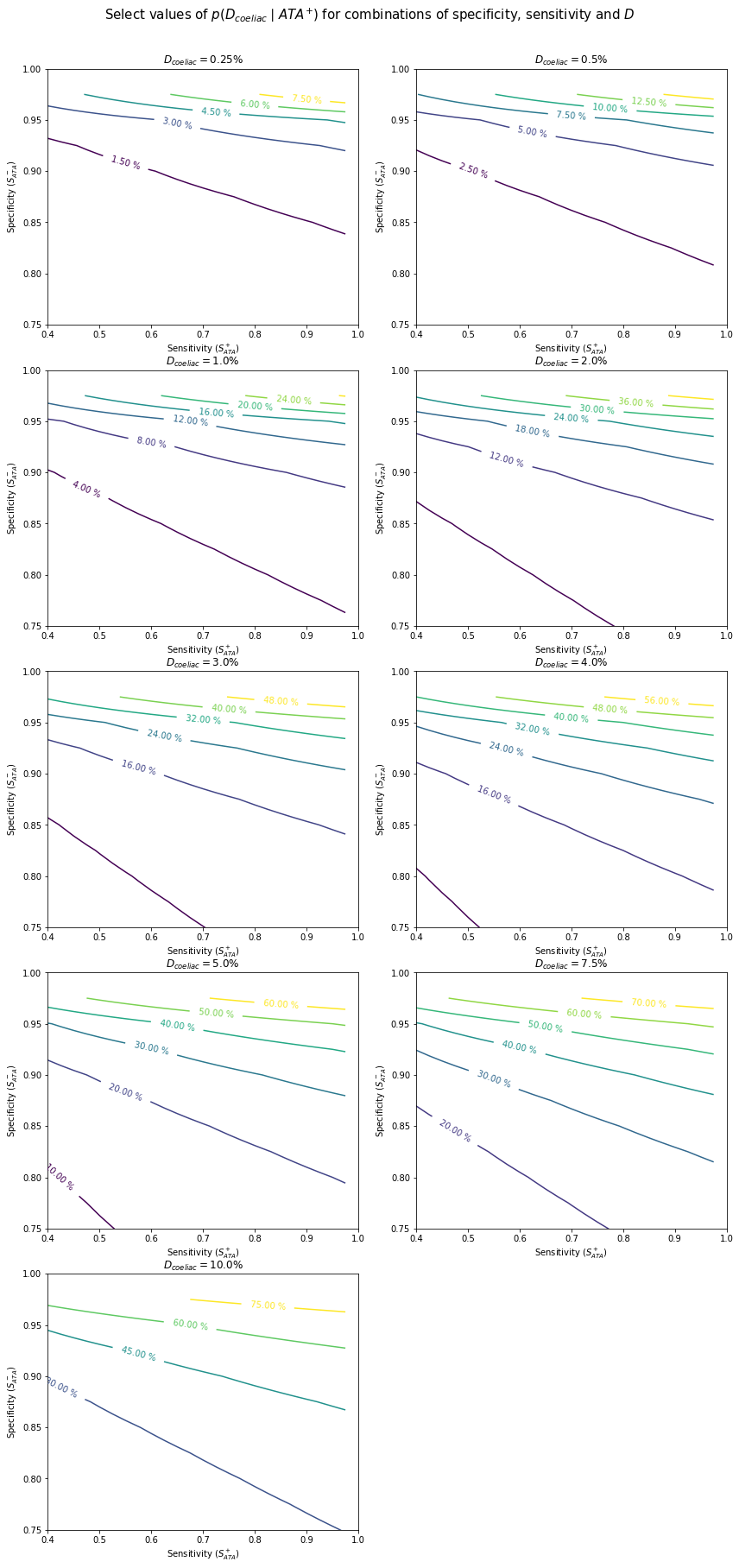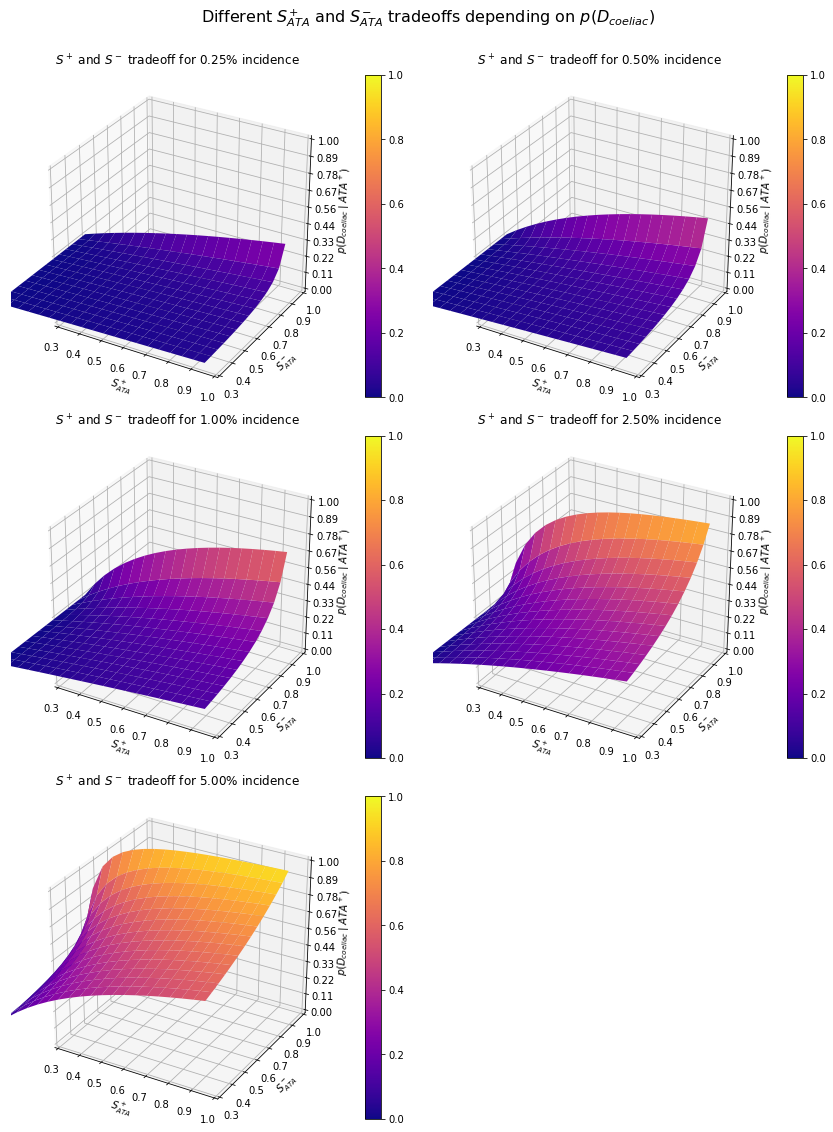# ATA testing example¶

There are several ways of testing for coeliac disease, a metabolic disorder in which the body responds to gluten proteins (gliadins and glutenins) in wheats, wheat hybrids, barley, oats and rye. One diagnostic approach looks at genetic markers in the HLA-DQ (Human Leukocyte Antigen type DQ), part of the MHC (Major Histocompatibility Complex) Class II receptor system. Genetic testing for a particular haplotype of the HLA-DQ2 gene, called DQ2.5, can lead to a diagnosis in most patients. Unfortunately, it's slow and expensive. Another test, a colonoscopic biopsy of the intestines, looks at the intestinal villi, short protrusions (about 1mm long) into the intestine, for tell-tale damage – but this test is unpleasant, possibly painful and costly.

So, a more frequent way is by looking for evidence of an autoantibody called anti-tissue transglutaminase antibody (ATA) – unrelated to this gene, sadly. ATA testing is cheap and cheerful, and relatively good, with a sensitivity ($Sˆ+_{ATA}$) of 85% and specificity ($Sˆ-_{ATA}$) of 97%.(Lock, R.J. et al. (1999). IgA anti-tissue transglutaminase as a diagnostic marker of gluten sensitive enteropathy. J Clin Pathol 52(4):274-7.) We also know the rough probability of a sample being from someone who actually has coeliac disease – for a referral lab, it's about 1%.

Let's consider the following case study. A patient gets tested for coeliac disease. Depending on whether the test is positive or negative, what are the chances she has coeliac disease?

## Setting up the constants¶

First, we need to set our seed variables, i.e. the variables we know be definition:

• $p(D_{coeliac})$ (p_coeliac): 0.01
• $S^+_{ATA} = p(+ | D_{coeliac})$ (ATA_sensitivity): 0.85
• $S^-_{ATA} = p(- | \neg D_{coeliac})$ (ATA_specificity): 0.97
In :
D_coeliac = 0.01
ATA_specificity = 0.97
ATA_sensitivity = 0.85


Because events are mutually exclusive ($p(E \mid \neg E) = 0$), we can express $p(\neg D_{coeliac})$ as $1 - p(D_{coeliac})$.

## ATA positive¶

If ATA is positive (event $ATA^+$), what's the likelihood the patient has coeliac disease (probability $p(D_{coeliac} \mid ATA^+)$)?

By Bayes' theorem, we get

$$p(D_{coeliac} \mid ATA^+) = \frac{p(ATA^+ \mid D_{coeliac}) \cdot p(D_{coeliac})}{p(ATA^+)}$$

Let's consider each term in isolation.

### $p(ATA^+)$¶

The value of $p(ATA^+)$ is the uncondtional probability of a positive test result, calculated as the sum of true and false positives, using specificity ($S^-_{ATA}$) and sensitivity ($S^+_{ATA}$).

$$p(ATA^+_{true}) = p(+ \mid D_{coeliac}) \cdot p(D_{coeliac}) = S^+_{ATA} \cdot p(D_{coeliac})$$$$p(ATA^+_{false}) = p(+ \mid D_{\neg coeliac}) \cdot p(D_{\neg coeliac}) = (1 - S^-_{ATA}) \cdot (1 - D_{coeliac})$$
In :
ATA_true_pos = ATA_sensitivity * D_coeliac
ATA_false_pos = (1 - ATA_specificity) * (1 - D_coeliac)


### $p(D_{coeliac})$¶

The value of $p(D_{coeliac})$ the known frequency of coeliac disease in the population examined, and set at 1% or $0.01$. In reality, the prevalence of coeliac disease in the population is approximately 1:400 or $0.0025$, but it's important to remember that the probability of the actual event has to necessarily pertain to the probability of the event as perceived at the point of analysis, in this case, at the lab. Purely statistically, the people referred to the lab are not a random sample from the population – they're referred to the lab for a reason, and that reason is that they show symptoms that might be coeliac disease. Bottom line – always know the base population.

## $p(ATA^+ \mid D_{coeliac})$¶

The conditional probability of $ATA^+$ given $D_{coeliac}$ comprises the cases when the patient has coeliac disease, and the test correctly detects it – in other words, the sensitivity $S^+_{ATA}$.

$$p(ATA^+ \mid D_{coeliac}) = p(+ \mid D_{coeliac}) = S^+_{ATA}$$

## $p(D_{coeliac} \mid ATA^{+})$¶

Per Bayes' theorem,

$$p(D_{coeliac} \mid ATA^{+}) = \frac{p(ATA^{+} \mid D_{coeliac}) \cdot p(D_{coeliac})}{p(ATA^{+})}$$

Expanding that, we get

$$p(D_{coeliac} \mid ATA^+) = \frac{S^+_{ATA} \cdot p(D_{coeliac})}{p(ATA^+_{true}) + p(ATA^+_{false})}$$
In :
p_coeliac_if_ATA_pos = ((ATA_sensitivity * D_coeliac)/(ATA_true_pos + ATA_false_pos))

print("The probability that a positive ATA test result came from a person who actually has coeliac disease is {coeliac_ATA:.2f}%.".format(coeliac_ATA = p_coeliac_if_ATA_pos * 100))

The probability that a positive ATA test result came from a person who actually has coeliac disease is 22.25%.


## Visualised...¶

One of the features is that incremental increases in sensitivity and specificity have unequal results. This is best seen when plotting them in a two-dimensional space for a fixed value of $D$. The contour plot shows the likelihood that a positive result will come from a patient with coeliac disease ($p(D_{coeliac} \mid ATA^+)$), dependent of specificity ($S^{-}_{ATA}$) and sensitivity ($S^{+}_{ATA}$), for a given proportion of coeliac disease in all samples ($D$ or $p(D_{coeliac})$).

In :
# First, we need to describe the relationship between the
# variables as a single function.

def ATA_sensitivity_specificity_function(sensitivity, specificity, D = 0.01):
true_pos = sensitivity * D
false_pos = (1 - specificity) * (1 - D)

return ((sensitivity * D) / (true_pos + false_pos))

In :
%matplotlib inline
from numpy import arange
from pylab import meshgrid, cm, imshow, contour, clabel, colorbar, axis, title, show
import matplotlib.pyplot as plt
import matplotlib.gridspec as gridspec
import numpy as np
from math import ceil

In :
D_values = (0.0025, 0.005, 0.01, 0.02, 0.03, 0.04, 0.05, 0.075, 0.1)

cols = 2
rows = ceil(len(D_values) / cols)
m_factor = 5

fig = plt.figure(figsize = (m_factor * cols + 2, m_factor * rows + 1.5))
fig.suptitle("Select values of $p(D_{coeliac}\mid ATA^{+})$ for combinations of specificity, sensitivity and $D$",
fontsize = 15)

for idx in range(rows):
for iidx in range(cols):
_idx = 2*idx + iidx

if _idx + 1 > len(D_values):
pass

else:

D_val = D_values[_idx]
ax = plt.subplot2grid((rows, cols), (idx, iidx))
box = ax.get_position()
ax.set_position([box.x0, box.y0, box.width, box.height * 0.95])

ax.set_title("$D_{coeliac} =$" + str(D_val * 100) + "%")

delta = 0.025
x, y = np.arange(0, 1, delta), np.arange(0, 1, delta)
X, Y = np.meshgrid(x, y)
Z = ATA_sensitivity_specificity_function(X, Y, D = D_val)

CS = ax.contour(X, Y, Z)
CS.levels = 100*CS.levels
CS.ax.set_xlim(0.4, 1)
CS.ax.set_ylim(0.75, 1)
CS.ax.set_ylabel("Specificity ($S^-_{ATA}$)")
CS.ax.set_xlabel("Sensitivity ($S^+_{ATA}$)")

if plt.rcParams["text.usetex"]:
fmt = r'%.2f \%%'
else:
fmt = '%.2f %%'
CS.ax.clabel(CS, CS.levels, inline = True, fmt = fmt, fontsize = 10)

plt.tight_layout(rect=[0, 0.03, 1, 0.95])
plt.show()In :
## To turn this into a D-conditional 3d plot, we can turn the X, Y and Z values into a 3D surface.
#
# Warning: this is gonna take a truckload of time if you set the resolution too high.

from mpl_toolkits.mplot3d import Axes3D
from matplotlib import cm
from matplotlib.ticker import LinearLocator, FormatStrFormatter
import matplotlib.pyplot as plt

######
# Define list of D-values here
######
D_values = (0.025, 0.05, 0.1, 0.25, 0.5)

# Define value ranges
sensitivity = arange(0.0, 0.99, 0.05)
specificity = arange(0.3, 0.99, 0.05)

# Define plot size
cols = 2
rows = ceil(len(D_values) / cols)
m_factor = 5

# Creates point mesh grid
X,Y = meshgrid(sensitivity, specificity)

# Create fig
fig = plt.figure(figsize = (m_factor * cols + 2, m_factor * rows + 1.5))
fig.suptitle("Different $S^{+}_{ATA}$ and $S^{-}_{ATA}$ tradeoffs depending on $p(D_{coeliac})$", fontsize = 16)

for idx in range(rows):
for iidx in range(cols):
_idx = 2*idx + iidx

if _idx + 1 > len(D_values):
pass

else:

D_val = D_values[_idx]
ax = plt.subplot2grid((rows, cols), (idx, iidx), projection = '3d')
box = ax.get_position()
ax.set_position([box.x0, box.y0 + 50, box.width, box.height * 0.95])

ax.set_title("$D_{coeliac} =$" + str(D_values[_idx] * 100) + "%")

Z = ATA_sensitivity_specificity_function(X, Y, D = D_values[_idx])

surf = ax.plot_surface(X, Y, Z,
rstride = 1, cstride = 1,
cmap = cm.plasma,
linewidth = 0,
antialiased = True,
vmin = 0, vmax = 1)

ax.zaxis.set_major_locator(LinearLocator(10))
ax.zaxis.set_major_formatter(FormatStrFormatter('%.02f'))
ax.invert_yaxis()
fig.colorbar(surf)
ax.set_xlabel("$S^+_{ATA}$")
ax.set_ylabel("$S^-_{ATA}$")
ax.set_zlabel("$p(D_{coeliac} \mid ATA^+)%$")
ax.set_zlim3d(0, 1)
ax.set_xlim(0.3, 1)
ax.set_ylim(0.3, 1)
ax.set_title("$S^+$ and $S^-$ tradeoff for {v:.2f}% incidence".format(v = 10*D_values[_idx]), y=1.1)

### SHOW PLOT
plt.tight_layout(rect=[0, 0.03, 1, 0.95])
plt.show()In [ ]: